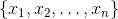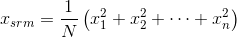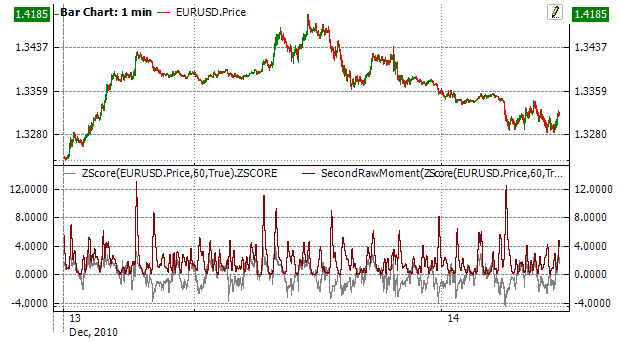﻿Second Raw Moment

# Second Raw Moment

The second raw moment (SecondRawMoment) (SRM) is defined as the mean square (the arithmetic mean of the squares of a set of numbers). The second raw moment is the square of the (RootMeanSquare).Calculation

In the case of a set of N values, the SRM isChart ExampleImplementation and Usage

To initialize indicator, use one of the following constructors:

SecondRawMoment – set default values: period = 14

SecondRawMoment(Int32) – set value for period

SecondRawMoment(TimeSpan) – sets time period

Use

SRM - property to get current valueExample
C#
``` 1// Create new instance
2var indicator = new SecondRawMoment(28);
3
4// Number of stored values
5indicator.HistoryCapacity = 2;
6